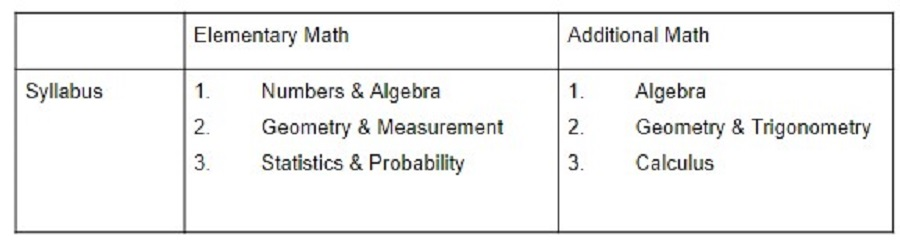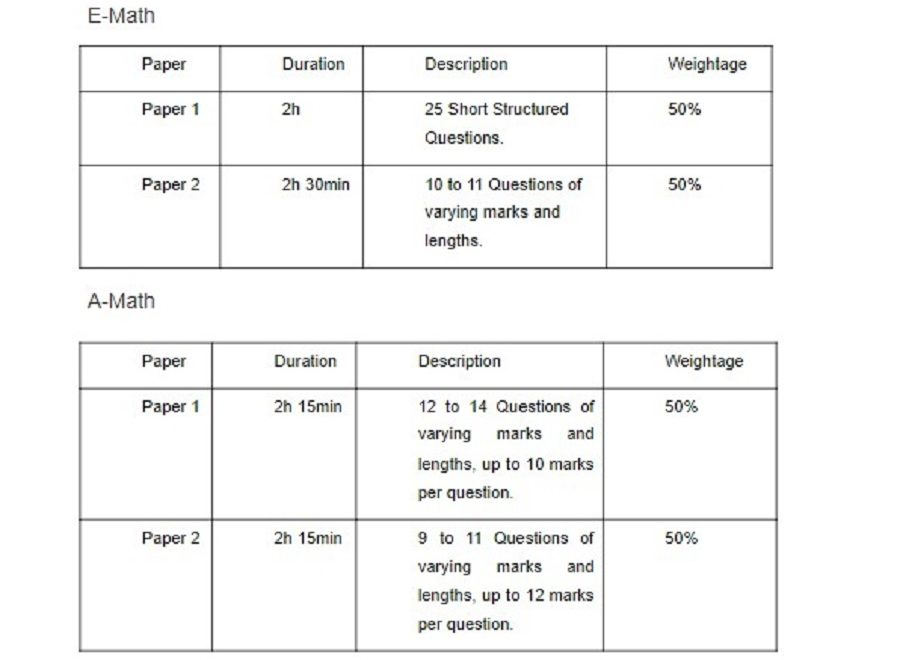# How is A-Math Different from E-Math?

## 28 Jun How is A-Math Different from E-Math?‘Should I take both E-Math and A-Math or just E-Math when I go on to Upper Secondary?’

‘Why do I need to take A-Math if I already plan to take E-Math?’

‘If I study A-Math, does it give me a better chance of entering the course I want in the future?’

If you are pondering the questions above with uncertainty, chances are you are now in the midst of making a very important decision concerning what subject combination you should take when you progress to Upper Secondary. Firstly, we would like to congratulate you on successfully clearing your Secondary 2 examinations. It is no small feat, you should be proud of yourself for the hard work you have put in! Soon, you will be leveling up to Secondary 3. Before you do, we hope to shed some light on the differences between E-Math and A-Math so that you will be better informed about which subject combination may work best for you.

### An Introduction to A Math & E Math

E-Math stands for Elementary Mathematics while A-Math stands for Additional Mathematics. Just by looking at the names, you can make an educated guess that E-Math will provide you  with the basics of Math, while A-Math will expose you to additional and more challenging Math concepts. The complexity level is what differentiates E-Math from A-Math, but  let us explore the aspects that set the two apart.

To give you an overview, the aim of E-math is to prepare you with fundamental math knowledge and skills, as well as to develop your ability to reason and form connections . A-Math, on the other hand, is higher studies in math. In A-Math, students build skills for analyzing and applying  deeper math concepts  in Algebra, Calculus, Geometry and Trigonometry to prepare them for  A-Level H2 Math or advanced mathematics in some modules in Polytechnics.

It is important to note that students who enroll in O-Level Additional Math will also be taking O-Level Elementary Math. The option of studying A-Math without studying E-Math is not available to students. Hence, it is compulsory for you to study E-Math, while A-Math is an optional course. So, the question to keep in mind as you read on is ‘Should I study A-Math?’.

### Comparing A-Math & E-Math SyllabusE-Math emphasizes the everyday application of Math and requires you to understand the meaning of common math terms like installments, interest and GST. In addition to this, you will be introduced to math tools that you can use in daily life, such as measurements and estimation. Thus, you are likely to immediately see how E-Math helps you in everyday life as soon as you learn it.

In contrast, it may take a while to realize how beneficial A-Math can be because it is more abstract. A-Math goes a little deeper to equip you with the research tools necessary for other fields of study, including Physics, Biology and Economics and many others.  For instance, how do we measure the rate of spread of COVID-19 in the community given a single infected person? Concepts taught in the topics of exponentials, logarithms and calculus allow a student to better understand the science and the research behind daily statistics and news reports.### Comparing A-Math & E-Math Assessment FormatIn terms of the mode of assessment, both A-Math and E-Math are similar in the sense that you will need to sit for two exam papers per subject. The weightage of each paper also accounts for 50% of the total marks, although the structure of the examination varies from paper to paper.

### Comparing the Complexity of A-Math & E-Math

Is A-Math more complex and harder to ace than E-Math? You might think A-Math is obviously more difficult than E-Math, however, the answer is not as straightforward as you may think. It all boils down to each individual and their preferences. Some students find A-Math more systematic. By memorizing math formulas and with sufficient practice, you will be able to score well in exams because the exam questions tend to be very similar to the questions you do for practice.  On the other hand, while most E-math questions are extensions of lower secondary math concepts, some questions can be rather creative and require one to flex their imaginations and logical faculties to arrive at the correct answer.  Some students  find the latter to be more challenging.

Moreover, the syllabus tested for A-Math is only from the content covered over the course of two years – Secondary 3 and Secondary 4. In comparison, the syllabus tested for E-Math is from the content covered over four years.

Some may feel E-Math concepts are easier to grasp since they are cumulative. Students have two years to build their E-Math foundation before moving on to Upper Secondary in which the questions gradually increase in complexity based on what they have learnt. This gives students more time to get used to the structure of E-Math questions. On the other hand, others find it easier to score for A-Math because they only need to retain the knowledge of two years of A-Math materials instead of four years’ worth of E-Math material.If you choose to study in a Junior College upon graduating from Secondary School, then further your studies in Engineering, Math or Science in University, it would be wise and beneficial that you opt for A-Math. This is simply because A-Level H2 Math is a prerequisite in most STEM courses in Universities, such as NTU and NUS.

If a student takes H2 Math in A-Level, there is a lot of assumed knowledge in A-Math, especially Calculus, Trigonometry and Logarithms. Some JCs may allow students to take H2 Math without an A-Math background, though they will be required to take a math bridging course to try to bring them up to speed with the rest of their peers. It is possible for a student who did not study A-Math to take H2 Math, however if they do not have a background in A-Math, it may be much harder to understand and do well in the subject. Students who did not study A-Math before will find Calculus, which makes up a significant portion of the H2 Math syllabus, challenging.

If you also wish to pursue a Polytechnic Diploma in an Engineering course, students are also introduced to advanced topics in math, such as Fourier and Laplace transforms, as well as Complex Analysis. Needless to say, a firm grounding in A-math will definitely make an easier time of your studies.

If you are uncertain about your aspirations for the future, we encourage you to start thinking about it now. Consult your teachers, parents and older siblings to get their perspectives and carefully consider what you want.